## 2. 4. 2 Association of Functions and Topological Elements

Once the simulation domain is tessellated, the topological structure of a finite cell complex is established, and a geometrical meaning is added to the cell complex, it has to be clarified, if such a structure is useful for the representation of functions. It is considered that all these elements can be handled in the computer and various values can be stored on different elements of the finite cell complex. In the following a method for the construction of a function space based on an existing cell complex is given.

First, the function is defined by choosing shape functions and what is further defined by the choice of weighting coefficients. It is clear that a tradeoff has to be found, because a too small number of shape functions leads to a bad representation of the solution function within the chosen function space and accordingly to a discretization error. Choosing too many shape functions makes the calculations consume too many resources and too much time.

A typical tradeoff is the choice of linear shape functions. The values which are obtained on the common points of the complex are defined explicitly (by value) whereas the shape of the functions is defined implicitly as linear on the single geometrical elements.

In the following it is assumed that each local shape function is transformed onto each cell which gives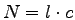basis functions of a function space, where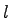denotes the number of local shape functions anddenotes the number of cells in the cell complex. Each basis function returns the value of the transformed local shape function within its cell of definition whereas it returns zero otherwise. Using this method, one obtains a function space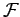spaned by shape functions which are defined on the simulation domain.

In order to exactly address one of the basis functions it is necessary to specify the cell where the basis function is defined. Moreover, the local shape function has to be given in order to uniquely specify a basis function. At this point it is convenient not to number the shape functions but to use the elements covering the collocation points of the basis function, which are called collocation elements and named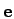.

As an example, the function spaceof cell-wise linear basis functions is considered. Each basis function of the function spacecan be specified by giving a cell as well as the collocation element. Each basis function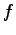of the function spacecan be written as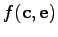.

Each shape function is directly assigned a weighting function that is associated with the same topological element as the respective shape function. For the function spaces with higher-order polynomial shape functions, the association of functions to topological elements can be performed in analogy. However, it has to be considered that if on single topological elements more shape functions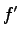are collocated, quantity vectors have to be used instead of scalar quantities.

In the following the question is discussed how the value of a function given as shown in the former section can be evaluated at a certain position. For storing a function within a computer, the following definitions, assumptions, and stored data have to be available as explained in the former sections.

• Topological cell complex
• Geometrical base shapes (implicit)
• Geometrical coefficients (explicit)
• Shape functions (implicit)
• Weighting coefficients (explicit)

Each function given as a linear combination of basis functions of the a function space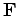can be written in the following manner.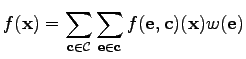(2.31)

When carrying out the summation by iterating all cells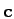and all incident collocation elementsalmost all elements are identically zero whereas only functions of the cell which holds the given point yield non-zero values.

A simplification can be obtained by determining the cell that holds the argument point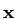. For this purpose several point search or point location algorithms  can be used. Both topological as well as geometrical properties have to be considered in order to find the cellwhich holds the given point. After the respective element has been found, the function has to be determined.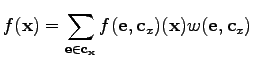(2.32)

whereis the cell that holds. The summation is carried out for all collocation elements. The basis function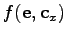is evaluated with the given pointas argument and is weighted with the function weight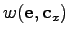.

Alternatively, the weight function can be used independently of the cellwhich restricts the function space and couples the weights that are associated with the same collocation element. It can be seen easily that the resulting function space is identical to the function space.

Finally, it can be stated that the definition of a function, comprises information about the weighting coefficients and the shape of the base functions. Information about the weighting coefficients requires memory for each single collocation element.

Information about the basis functions comprises the geometrical shape of the elements, the shape functions locally defined on the elements as well as the coefficients for the geometrical representation of the elements such as coordinates and curvature information.

If the number of collocation elements is increased by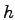-refinement , for instance doubled, the required memory for the weighting coefficients as well as the memory for storing the geometrical coefficients is doubled. Each newly added collocation element is assigned a weighting coefficient and, if necessary, geometrical information.

Adding a new function on the same topological structure (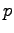-refinement) the memory required for weighting coefficients is increased by the number of elements, whereas memory usage for the geometrical coefficients remains unchanged.

Michael 2008-01-16